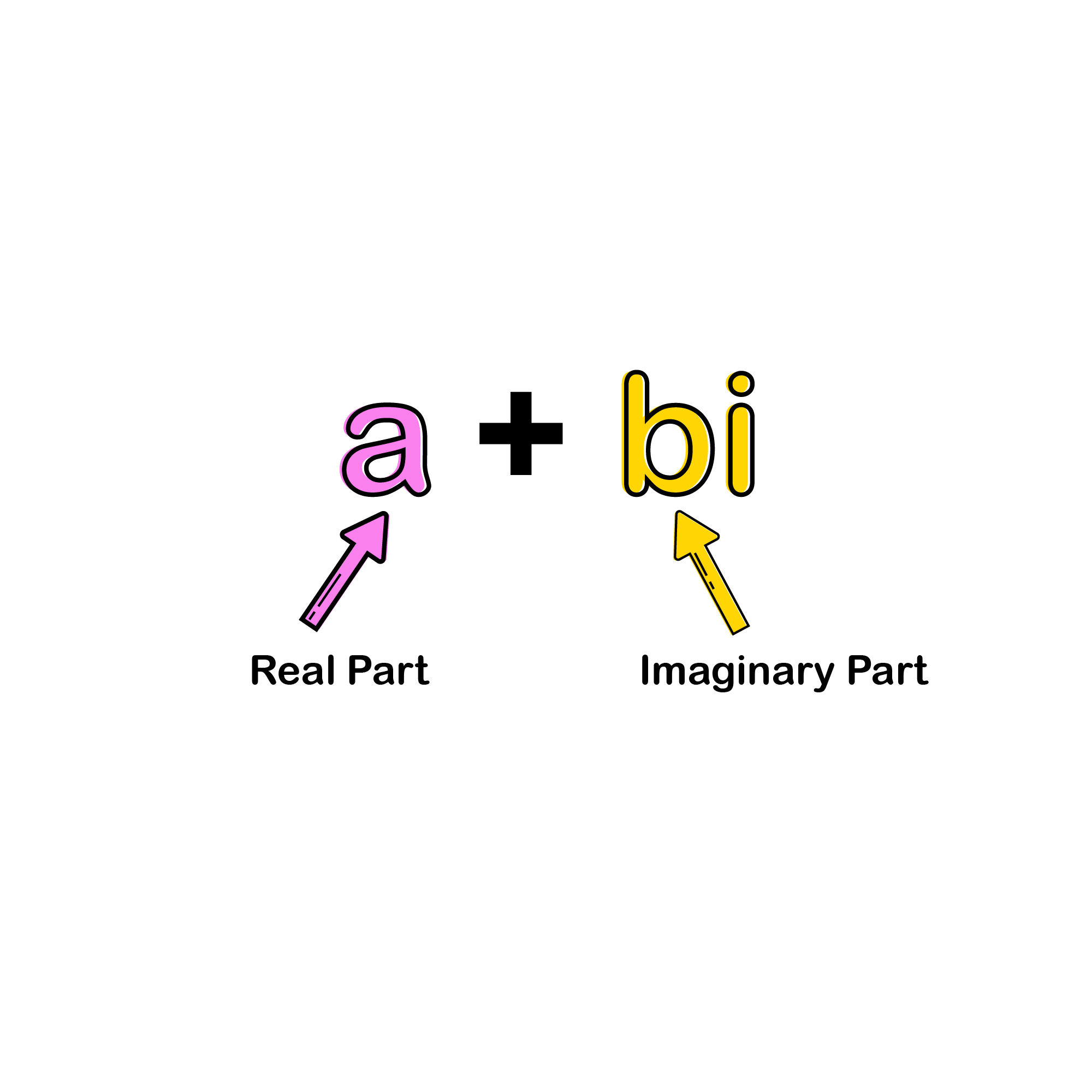Complex Numbers

# Complex NumbersThere is a new “number” that we associate negative numbers with under the square root. These are called COMPLEX NUMBERS, and they are indicated with the letter i, where: $$i=\sqrt{-1}$$

We can do regular operations like addition, subtraction, multiplication, and division with complex numbers. Keep the following in mind, to make complex multiplication simpler.

$$i=\sqrt{-1}$$
$$i^{2}=i\times i=\sqrt{-1}\times \sqrt{-1}=-1$$
$$i^{3}=i\times i\times i=\sqrt{-1}\times \sqrt{-1}\times \sqrt{-1}= -1\times \sqrt{-1}=-i$$
$$i^{4}=i^{2}\times i^{2}=-1\times -1=1$$
$$i^{5}=i^{4}\times i=i$$
$$i^{6}=i^{4}\times i^{2}=-1$$
$$i^{7}=i^{4}\times i^{3}=-i$$
$$i^{8}=i^{4}\times i^{4}=1$$

Solved Example 1
Find the value of $$i^{203}$$
A. i
B. -i
C. 1
D. -1

Solution
: Now, we need to first break up $$i^{203}\: to\: i^{202+1}$$
$$i^{202}\times i^{1}$$
$$(i^{2})^{101}\times i$$
$$(-1)^{101}\times i$$
-1 x i
= -i

### Add and Subtract Complex Numbers

Adding and subtracting complex numbers is similar to adding and subtracting polynomials. Just add or subtract the real and imaginary parts.

Example: Solve: (-6 - 5i) - (3 - 4i)
(-6 - 5i) - (3 - 4i) = (-6 - 5i) + (-3 + 4i)
= [-6 + (-3)] + (-5 + 4)i
= -9 - i

### Multiply Complex Numbers

Multiplying complex numbers is similar to multiplying polynomials.

Solve: (2 + 5i) (6 + 4i)
(2 + 5i) (6 + 4i) = 12 + 8i + 30i + 20i2
= 12 + 38i + 20(-1)
= 12 + 38i - 20
= -8 + 38i

### Divide Complex Numbers

For complex division, we need to know what a conjugate is. For starters, all complex numbers take the form of a + bi, where a is the x-coordinate and b is the y-coordinate. A complex conjugate is changing the + in a + bi to a − bi or vice versa. When we have complex division, we multiply by a clever form of “one,” which will be the conjugate of the denominator.

Example: Write each quotient in the form $$a+bi:\frac{6}{7-4i}$$

$$\frac{6}{7-4i}=\frac{6}{7-4i}\times \frac{7+4i}{7+4i}$$

$$\frac{42+24i}{49-16i^{2}}$$

$$\frac{42+24i}{49-16(-1)}$$

$$\frac{42+24i}{65}$$

$$\frac{42}{65}+\frac{25}{65}i$$## More Topics